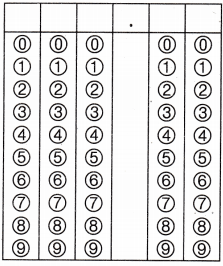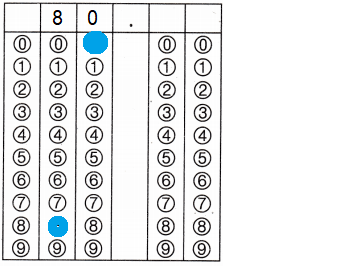Refer to our Texas Go Math Grade 4 Answer Key Pdf to score good marks in the exams. Test yourself by practicing the problems from Texas Go Math Grade 4 Module 12 Assessment Answer Key.

Vocabulary

• area
• pattern
• formula
• perimeter
• square unit (sq un)

Choose the best term from the box.

Question 1.
A ____________ is an ordered set of numbers or objects.
Pattern
Explanation:
Math patterns are sequences that repeat based on a rule, and a rule is a set way to calculate or solve a problem.

Question 2.
A set of symbols that expresses a mathematical rule is called a _____________ .
Formula
Explanation:
A set of symbols that expresses a mathematical rule or principle;
for example, the formula for the area of a rectangle is a = l x w,
where a is the area, l the length, and w the width.

Question 3.
The _____________ is the distance around a figure.
Perimeter
Explanation:
The perimeter of a two-dimensional figure is the distance covered around it.
It defines the length of shape, whether it is a triangle, square, rectangle or a circle.

Concepts and Skills

Use the rule to write the first six terms in the pattern.
Describe another pattern in the numbers.

Question 4.
Rule: Add 10.             First term: 11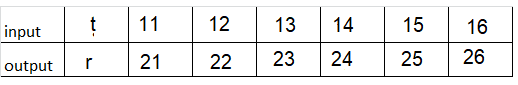Explanation:
Rule: Add 10.             First term: 11
t = 11,12,13,14,15,16
Input = t + 10
Output = 11 + 10 = 21
Input = t + 10
Output = 12 + 10 = 22
Input = t + 10
Output = 13 + 10 = 23
Input = t + 10
Output = 14 + 10 = 24
Input = t + 10
Output = 15 + 10 = 25
Input = t + 10
Output = 16 + 10 = 26

Use the rule to complete the input/output table.

Question 5.
Rule: The output is t – 3.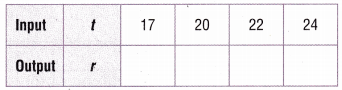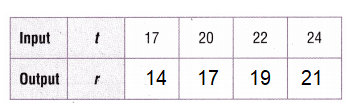Explanation:
Rule: The output is t – 3.
Input t = 17,20,22,24
Output r = t – 3
Question 6.
Rule: The output is a + 7.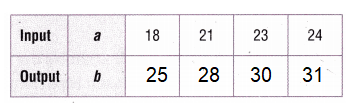Explanation:
Rule: The output is a + 7.
input a = 18 , 21, 23, 24
Output b = a + 7
Output b = 18 + 7 = 25
Output b = 21 + 7 = 28
Output b = 23 + 7 = 30
Output b = 24 + 7 = 31

Find the perimeter and area of the rectangle or square.

Question 7.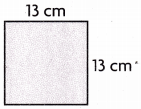Perimeter = 54 cm
Area = 169 cm
Explanation:
Perimeter of a Square = 4 × side
= 4 x 13 = 54 cm
Area of a Square = side × side
= 13 x 13 = 169 cm

Question 8.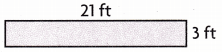Perimeter = 48 feet
Area = 63 feet
Explanation:
Length = 3 feet; Width = 21 feet
Area of a rectangle = l × w
= 3 x 21 = 63 feet
Perimeter of a rectangle = 2 × ( l + w )
= 2 x (3 + 21)
= 2 x 24 = 48 feet

Question 9.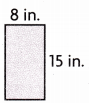Perimeter = 46 in
Area = 12 in
Explanation:
Length = 15 in ; Width = 8 in
Area of a rectangle = l × w
= 15 x 8 = 120 in
Perimeter of a rectangle = 2 × ( l + w )
= 2 x (15 + 8)
= 2 x 23 = 46 in

Question 10.
Erica knits 18 squares on Monday. She knits 7 more squares each day for the rest of the week. How many squares does Erica have after Friday?
(A) 39
(B) 90
(C) 53
(D) 46
Option (C)
Explanation:
Erica knits 18 squares on Monday.
She knits 7 more squares each day for the rest of the week.
Total squares does Erica have knits after Friday is 46 + 7 = 53
Rule : Add 7   First term is 18
Input = a + 7
Output b = a + 7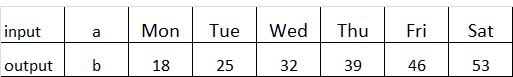Question 11.
Darren wants to use a formula to find the perimeter of the figure below. Which formula should Darren use?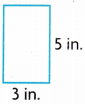(A) P = l + w
(B) P = l + w + l + w
(C) p = l × w
(D) p = 2 x l + w + l
Option(B)
Explanation:
The figure below Darren is in rectangle.
Perimeter of a rectangle = length + width + length + width
l = length; w = width

Question 12.
Carol wants to find the area of her rectangular garden below so she will know how much mulch to buy. Which formula should she use to find the area?(A) A = l + w
(B) A = 2 × w
(C) A = l × w + l × w
(D) A = l × w
Option(D)
Carol wants to find the area of her rectangular garden.
Area of a rectangle = l × w
length = 6 cm; width = 13 cm
A = 6 x 13 = 78 cm

Question 13.
The input-output table below shows the number of boxes, b, and the number of pillows, p, Jason is packing in the boxes. Using the rule b × 4, how many pillows would Jason pack in 7 boxes?Record your answer and fill in the bubbles on the grid. Be sure to use the correct place value.